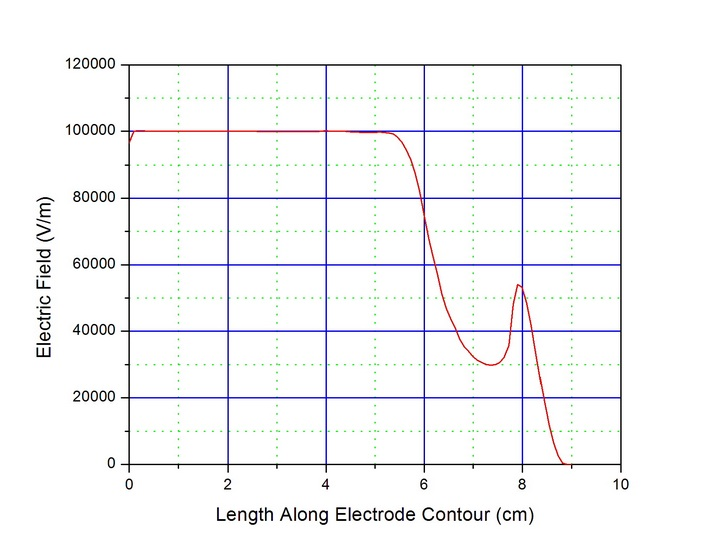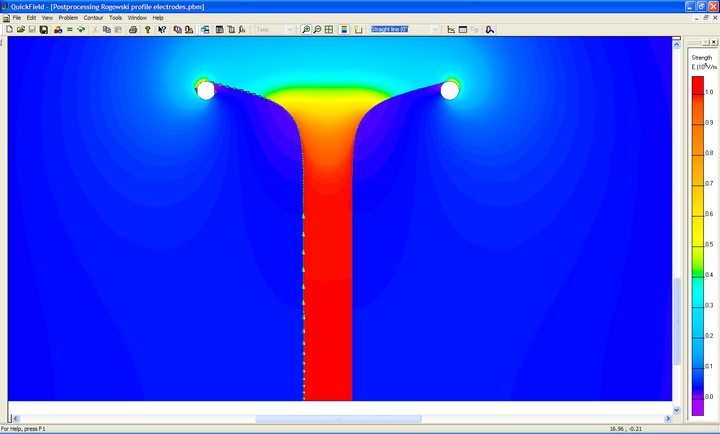# Rogowski Profile Electrodes

In many cases, it is desirable to produce uniform electric fields between a set of electrodes. However, simple parallel plate electrodes are finite in size and therefore have electric field enhancement at the edges of the plates. Several individuals, including Rogowski, have addressed these problems in axially symmetric geometries by defining equations for electrode profiles which avoid these issues by gradually decreasing the radius of curvature so that the fields at the edges do not exceed those values in the center of the electrodes. Rogowski extended the work done by Maxwell in deriving a solution for the electric fields associated with a finite flat plane above an infinite flat plane. Shown below is the model of a high voltage finite plate above an infinite ground plane.

The plot below reveals the electric fields associated with this geometry. In this case, the finite plate has a potential of 1000 V on it and the distance between it and the ground plane is 1 cm. As a result, the field amplitude in the homogenous region between the two is 100 kV/m. One can see that the fields at the termination of the high voltage plate are much higher (in this case, over 700 kV/m).

Shown in the following figure are the equipotential lines associated with this same geometry. In this case, each line represents 100 V.Equipotential Lines Associated With Finite Plate Above Infinite Plate

Maxwell’s analysis of the fields for the finite plate above the infinite plane are expressed by the equations below:

where psi represents equipotential lines or surfaces and phi are lines of electrostatic force. Both of these may take on various constant values. X and Y are the coordinates, a is the separation between the finite plate and infinite plane, and A=a/pi. Given a desired value of a, the field is calculated and plotted by assuming a value of psi and solving the equations for X and Y for a series of values of phi. Rengier found experimentally that electrodes constructed with psi values of pi/2 (0.5 pi) and 2pi/3 (0.66 pi) produced sparking always within the plane portion of the electrodes (no arcing happened at the edges). As a result, the pi/2 solution is a common one and for this special case, the equations shown above reduce to the following:

The figure below shows a set of (psi=pi/2) Rogowski electrodes which are symmetrical when rotated about the horizontal axis.

Shown below is the voltage potential plot for the electrodes shown above. In this case, 1000 V has been applied across the electrodes and the gap spacing between the two electrodes is 1 cm.

The plot below is the electric fields for the electrodes shown above. With 1000 V across the electrodes and the electrode gap spacing of 1 cm, the field amplitude between the vertical portions of the electrodes is 100 kV/m. As one can see, there is no field enhancement at the termination of the electrodes where they are radiused away from the plane sections. The fields at the electrode edges are no higher in amplitude than the fields in the main portion of the electrodes where the fields are uniform. The electrode profile is eventually terminated in a toroidal shape once the fields have decreased to a reasonable level. At that point, there is some additional field enhancement above what the fields were on the profile; however, they are still well below the nominal field levels between the parallel plane section of the electrodes.

The graph below is the amplitude of the electric field along the contour of the electrodes from the bottom to the top of the electrodes all the way through the radiused section and around the toroidal termination. As one can see, the fields decay away from the maximum value between the plan parallel section of the electrodes as they are radiused away. The fields at the toroidal termination are slightly higher in amplitude than the fields on the radiused section of the electrodes; however they are still less than 60% of the maximum Efield value.Electric Field Plot Along Contour of Rogowski Pi/2 Electrode Profile

The figure below shows the contour along the left electrode from the bottom (in the center of the parallel plane section of the electrodes) to the top of the electrodes.Electric Field Plot Showing Contour Along Rogowski Pi/2 Electrode Profile

A number of other electrode profiles have been developed over the years including profiles by Bruce, Ernst, Chang, Harrison, etc. Listed below are a number of technical references regarding uniform field electrode shapes including the original papers by Rogowski as well as those by Ernst, Chang, etc.

W. Rogowski, Arch. f. Elekt., Vol. 12, p. 1, 1923.
W. Rogowski, Arch. f. Elekt., Vol. 16, p. 73, 1926.
W. Rogowski, Arch. f. Elekt., Vol. 16, p. 76, 1926.
F.M. Bruce, J. Inst. Electr. Eng., Vol. 94, p. 138, 1947.
J.A. Harrison, Br. J. Appl. Phys., Vol. 18, p. 1617, 1967.
“Compact Uniform Field Electrode Profiles”, G. Ernst, Optics Communications, Vol. 47, No. 1, pp. 47-51, August, 1983.
“Uniform-Field Electrodes with Minimum Width”, G. Ernst, Optics Communications, Vol. 49, No. 4, pp. 275-277, March, 1984.
“Improved Uniform-Field Electrode Profiles for TEA Laser and High-Voltage Applications”, T.Y. Chang, The Review of Scientific Instruments”, Vol. 44, No. 4, pp. 405-407, April, 1973.
“A Uniform Field Electrode for Use in a Discharge Chamber of Restricted Size: Design and Performance”, J. Pearson and J. Harrison, Brit. J. Appl. Phys., Ser. 2, Vol. 2, pp. 77-84, 1969.
“A Compacted Ernst-Electrodes Profile for Pulsed High-Pressure Lasers”, I. Leyva and J. Guerra, Meas. Sci. Tech., Vol. 10, pp. N1-N2, 1999.
“A Novel Analytical Design Method for Discharge Electrode Profiles”, E. Stapperts, Appl. Phys. Letters, Vol. 40, No. 12, pp. 1018-1019, June, 1982.
“Gaseous Conductors – Theory and Engineering Applications”, J. Cobine, Dover Publications, pp. 177-181, 1958.

Send consulting inquiries, comments, and suggestions to richard.ness@nessengr.com